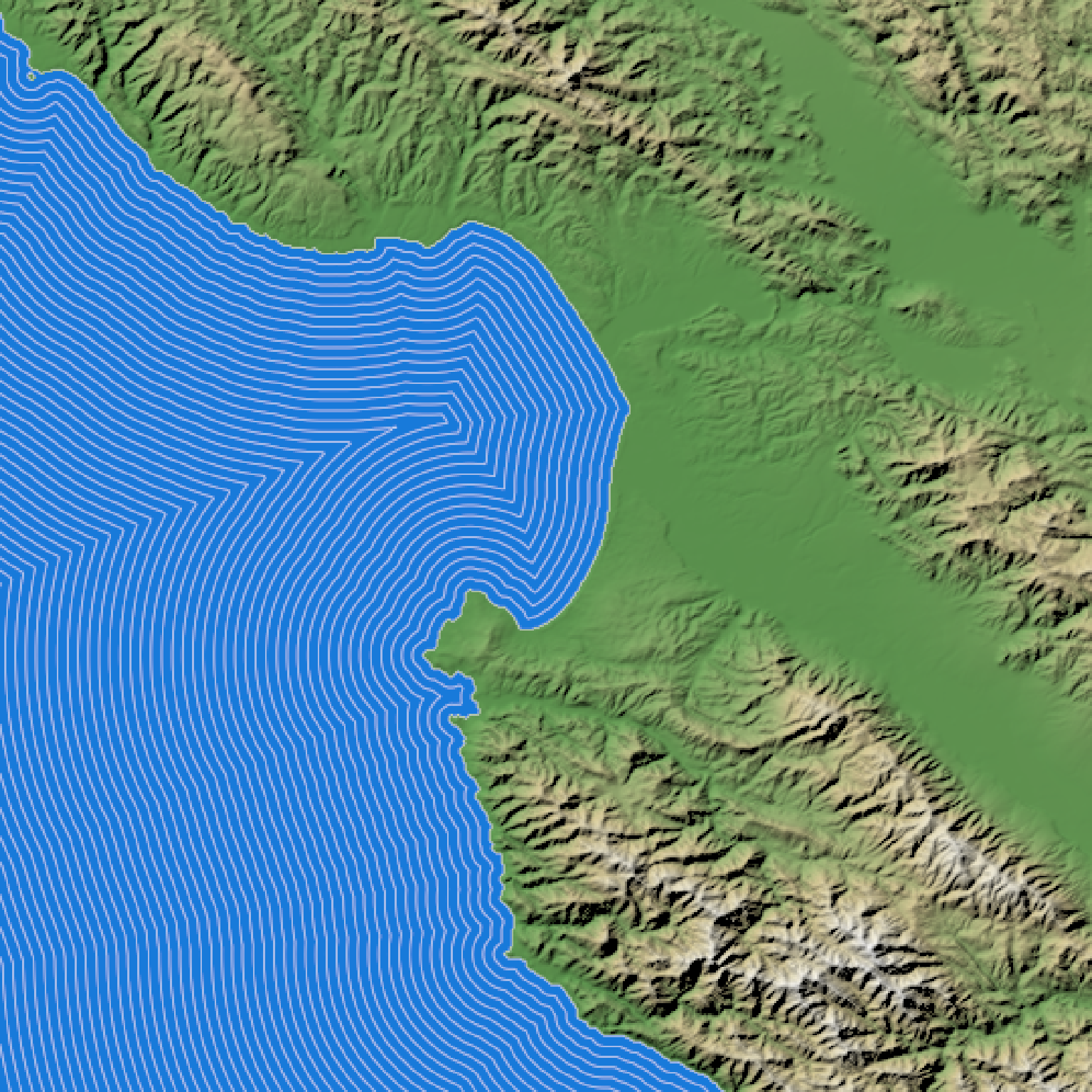Using a height map or a boolean matrix, generates a semi-transparent waterline overlay to layer onto an existing map. This uses the method described by P. Felzenszwalb & D. Huttenlocher in "Distance Transforms of Sampled Functions" (Theory of Computing, Vol. 8, No. 19, September 2012) to calculate the distance to the coast. This distance matrix can be returned directly by setting the return_distance_matrix argument to TRUE.

generate_waterline_overlay(
heightmap,
color = "white",
linewidth = 1,
boolean = FALSE,
min = 0.001,
max = 0.2,
breaks = 9,
smooth = 0,
alpha_dist = max,
alpha = 1,
falloff = 1.3,
evenly_spaced = FALSE,
zscale = 1,
cutoff = 0.999,
min_area = length(heightmap)/400,
max_height = NULL,
return_distance_matrix = FALSE
)

## Arguments

heightmap

A two-dimensional matrix, where each entry in the matrix is the elevation at that point. If boolean = TRUE, this will instead be interpreted as a logical matrix indicating areas of water.

color

Default white. Color of the lines.

linewidth

Default 1. Line width.

boolean

Default FALSE. If TRUE, this is a boolean matrix (0 and 1) indicating contiguous areas in which the lines are generated (instead of a height matrix, from which the boolean matrix is derived using detect_water())

min

Default 0.001. Percent distance (measured from the furthest point from shore) where the waterlines stop.

max

Default 0.2. Percent distance (measured from the furthest point from shore) where the waterlines begin.

breaks

Default 9. Number of water lines.

smooth

Default 0, no smoothing. Increase this to smooth water lines around corners.

Default TRUE. If FALSE, lines will not fade with distance from shore.

alpha_dist

Default to the value specified in max. Percent distance (measured from the furthest point from shore) where the waterlines fade entirely, when fade = TRUE.

alpha

Default 1. Maximum transparency for waterlines. This scales the transparency for all other levels.

falloff

Default 1.3. Multiplicative decrease in distance between each waterline level.

evenly_spaced

Default FALSE. If TRUE, falloff will be ignored and the lines will be evenly spaced.

zscale

Default 1. Arguments passed to detect_water(). Ignored if boolean = TRUE. The ratio between the x and y spacing (which are assumed to be equal) and the z axis. For example, if the elevation levels are in units of 1 meter and the grid values are separated by 10 meters, zscale would be 10.

cutoff

Default 0.999. Arguments passed to detect_water(). Ignored if boolean = TRUE.The lower limit of the z-component of the unit normal vector to be classified as water.

min_area

Default length(heightmap)/400. Arguments passed to detect_water(). Ignored if boolean = TRUE. Minimum area (in units of the height matrix x and y spacing) to be considered a body of water.

max_height

Default NULL. Arguments passed to detect_water(). Ignored if boolean = TRUE. If passed, this number will specify the maximum height a point can be considered to be water. FALSE, the direction will be reversed.

return_distance_matrix

Default FALSE. If TRUE, this function will return the boolean distance matrix instead of contour lines.

## Value

4-layer RGB array representing the waterline overlay.

## Examples

if(run_documentation()) {
#Create a flat body of water for Monterey Bay
montbay = montereybay
montbay[montbay < 0] = 0

#Generate base map with no lines
basemap = montbay %>%

plot_map(basemap)
}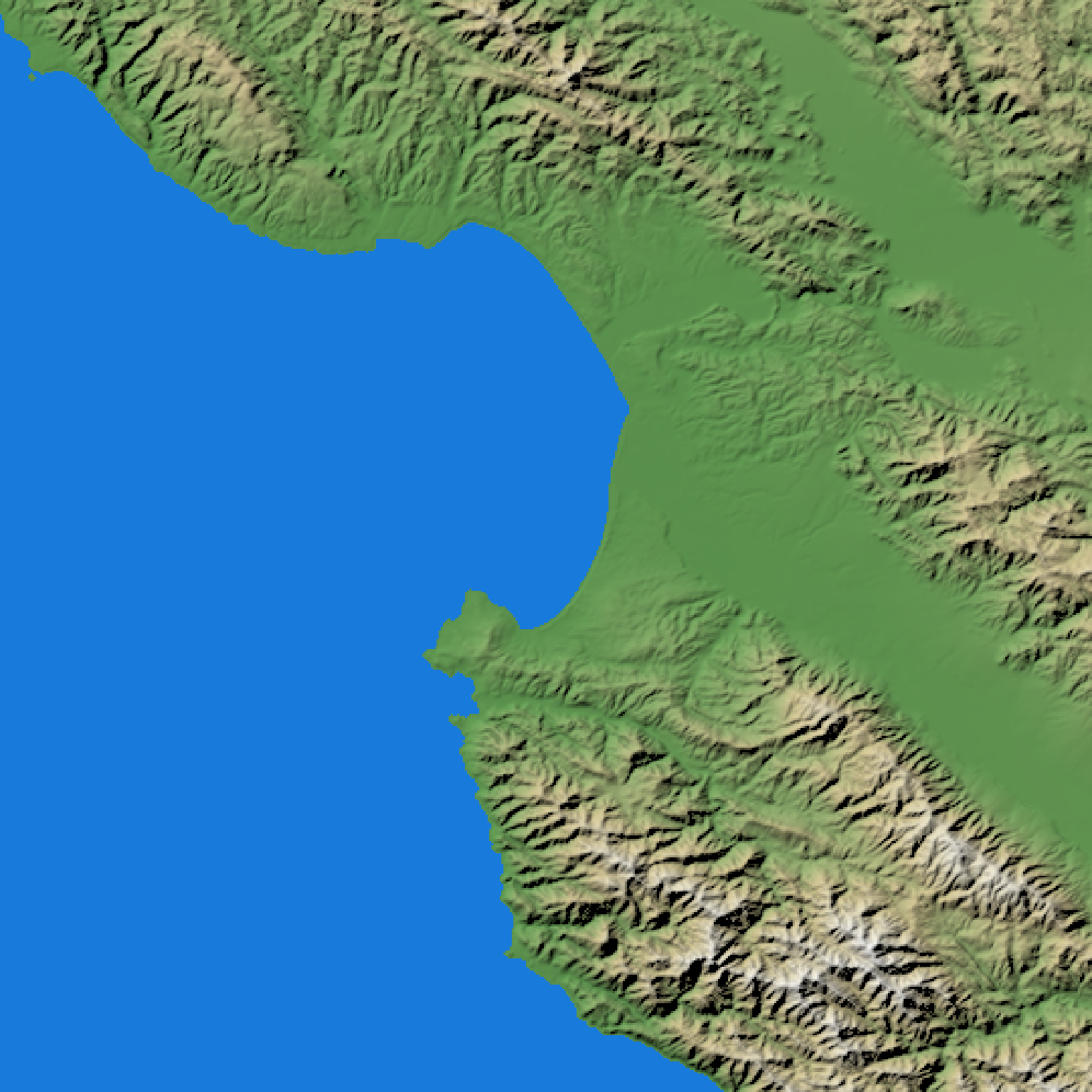if(run_documentation()) {
basemap %>%
plot_map()
}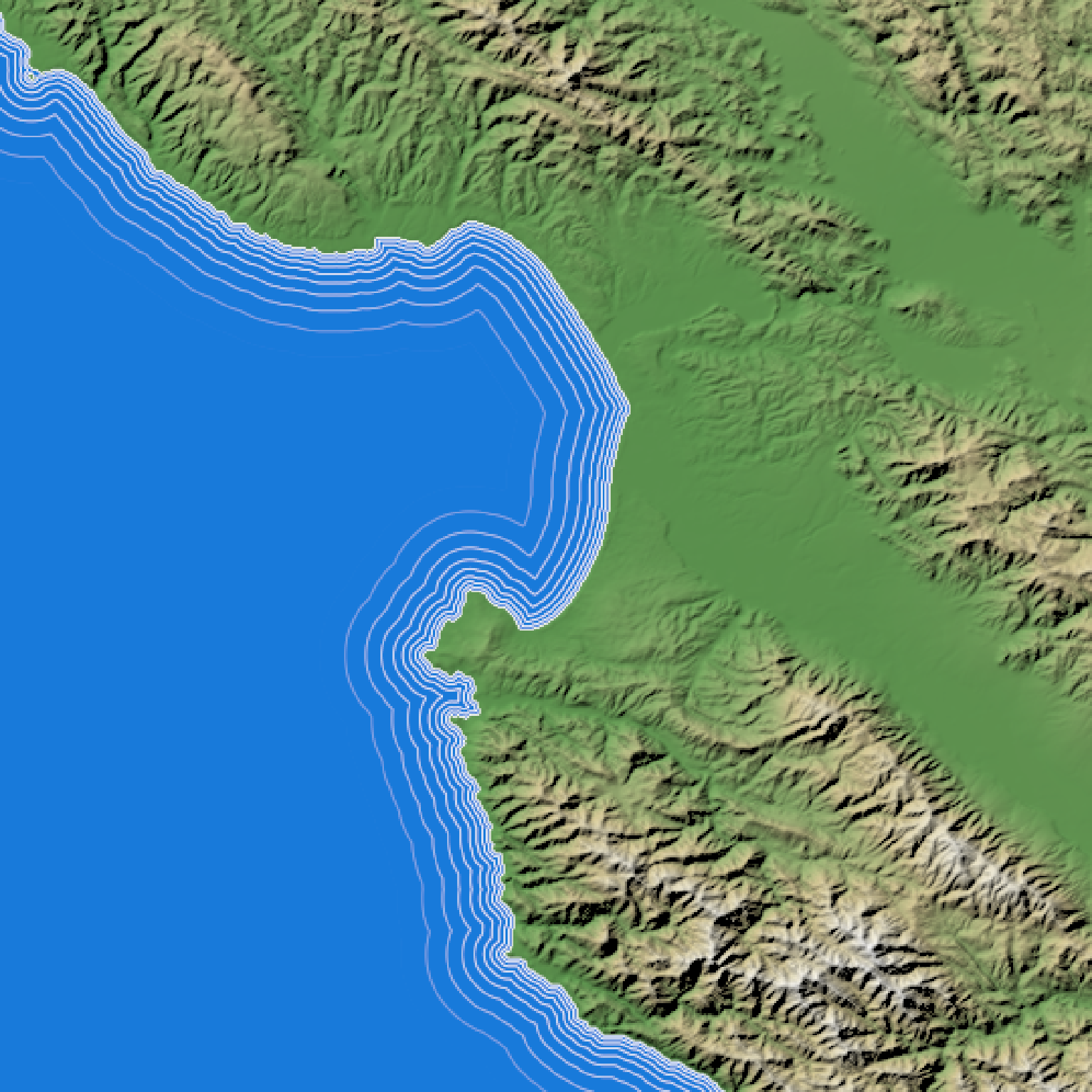if(run_documentation()) {
#Change minimum line distance:
basemap %>%
plot_map()
}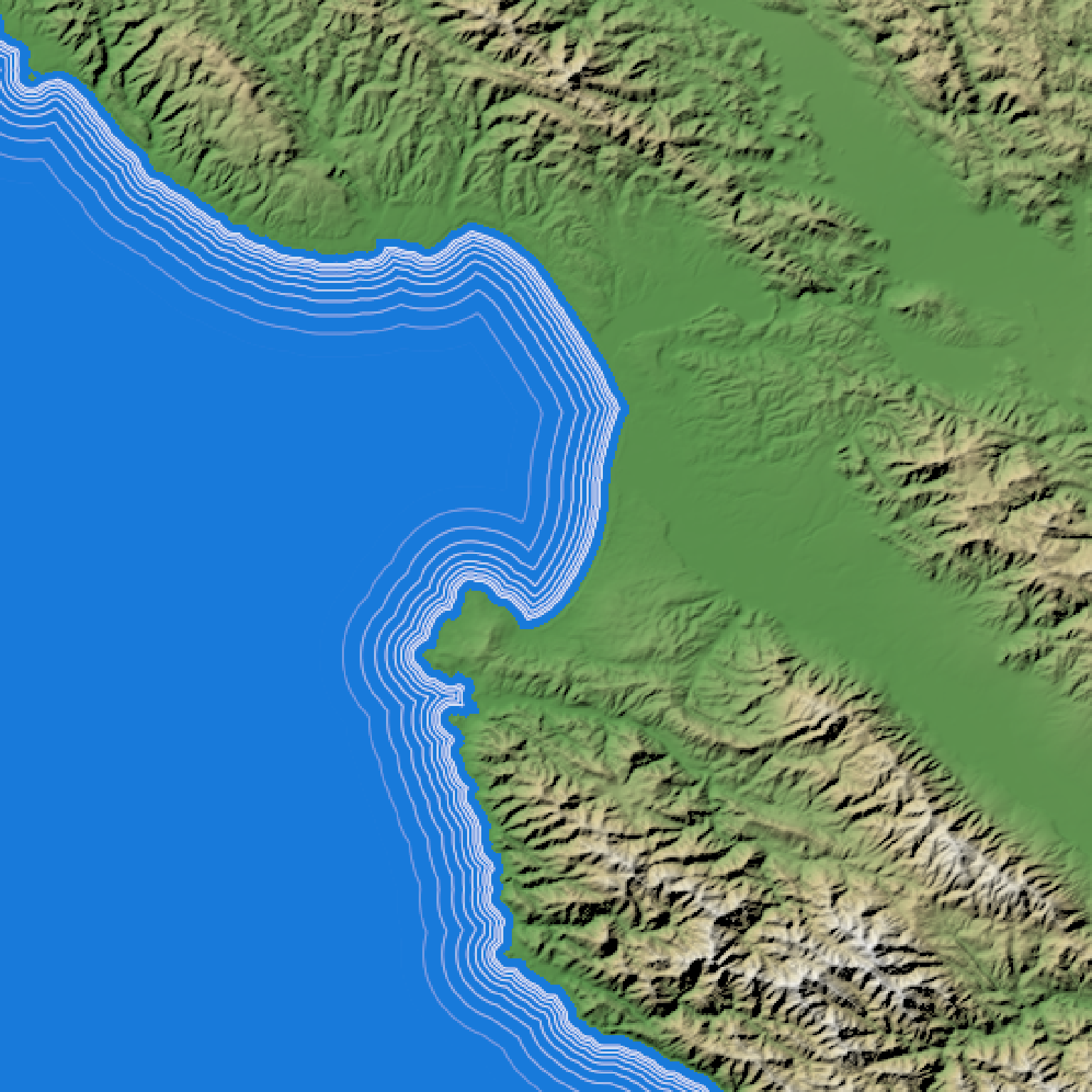if(run_documentation()) {
#Change maximum line distance
basemap %>%
plot_map()
}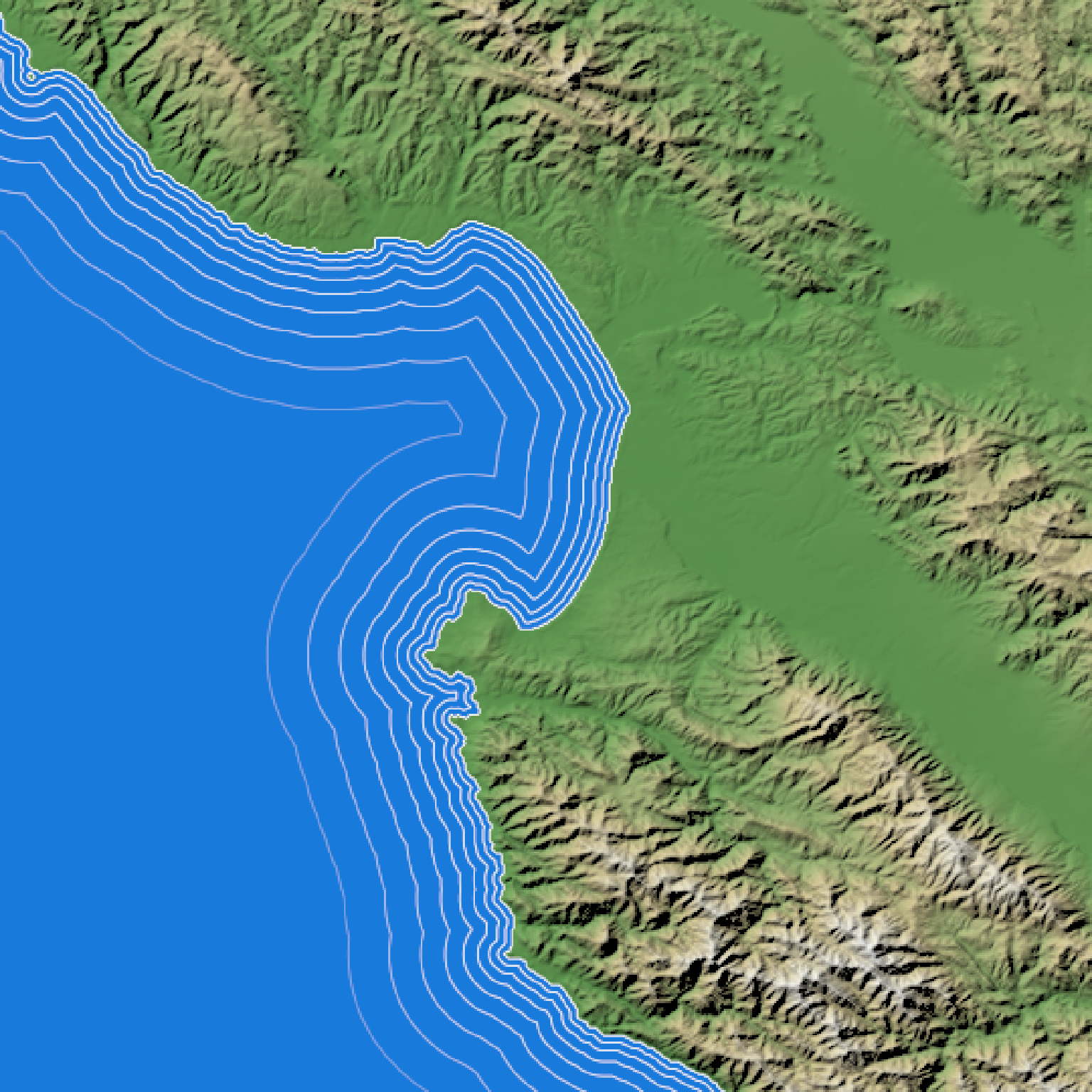if(run_documentation()) {
#Smooth waterlines
basemap %>%
add_overlay(generate_waterline_overlay(montbay, max = 0.4, smooth=2)) %>%
plot_map()
}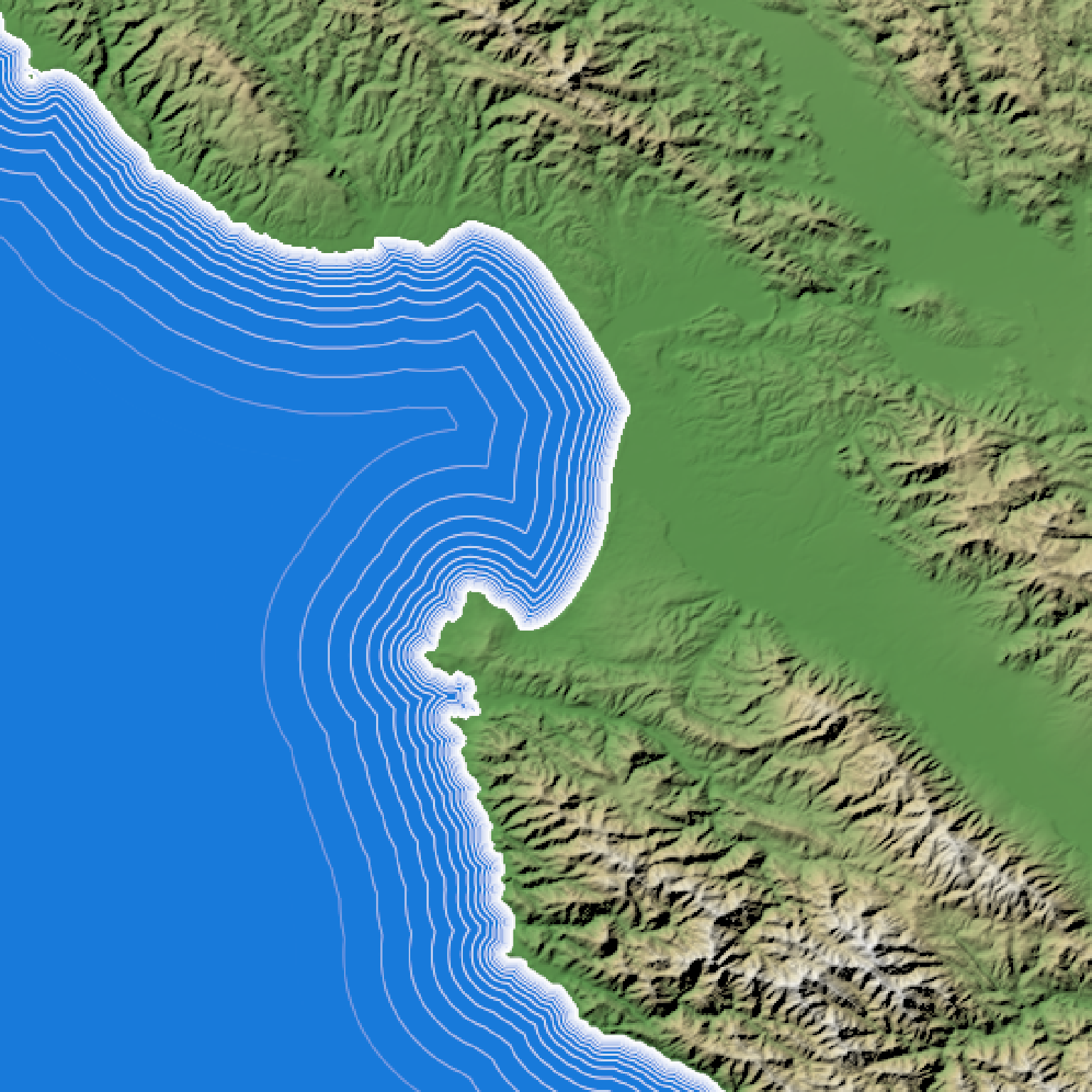if(run_documentation()) {
#Increase number of breaks
basemap %>%
add_overlay(generate_waterline_overlay(montbay, breaks = 20, max=0.4)) %>%
plot_map()
}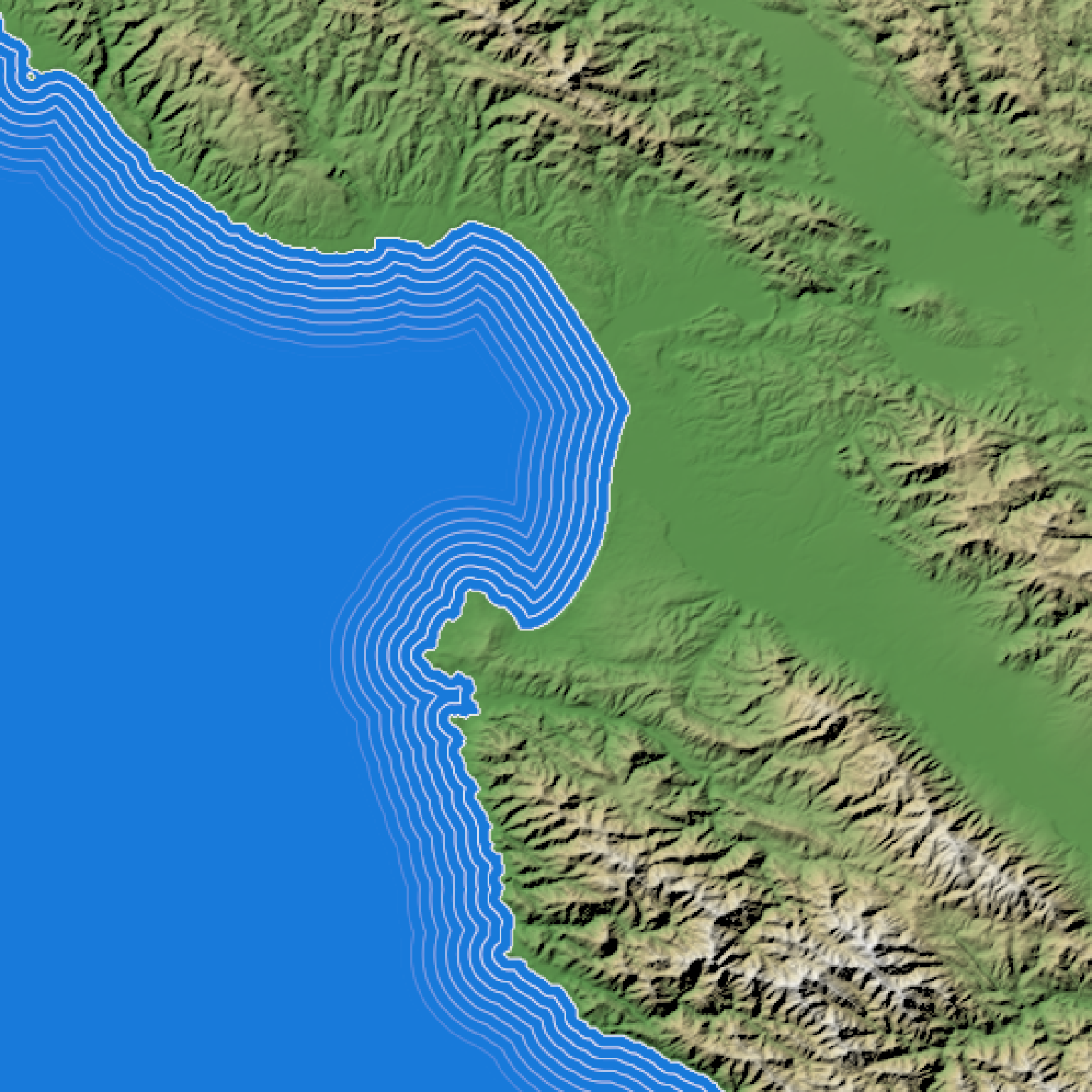if(run_documentation()) {
#Make lines evenly spaced:
basemap %>%
plot_map()
}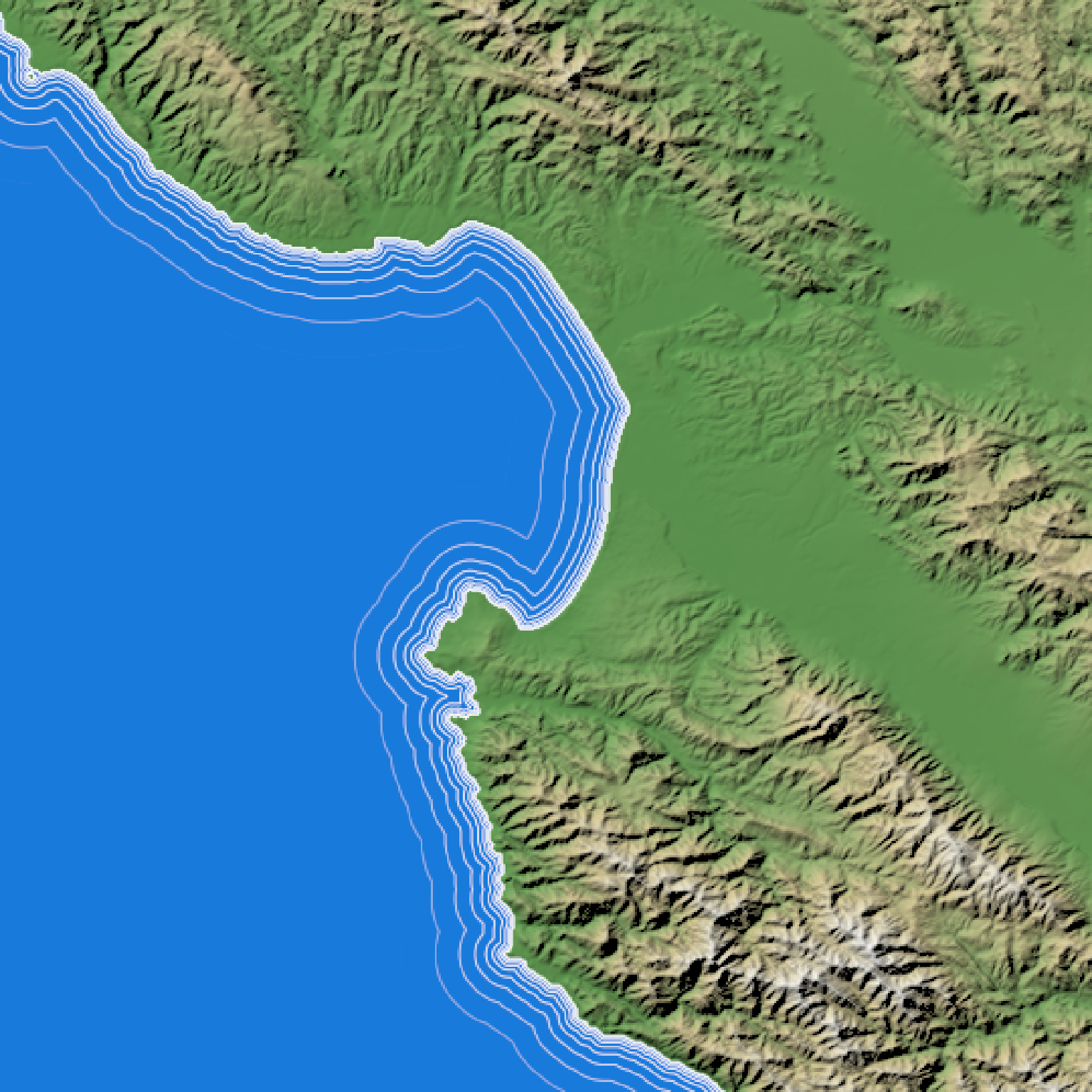if(run_documentation()) {
#Change variable distance between each line
basemap %>%
plot_map()
}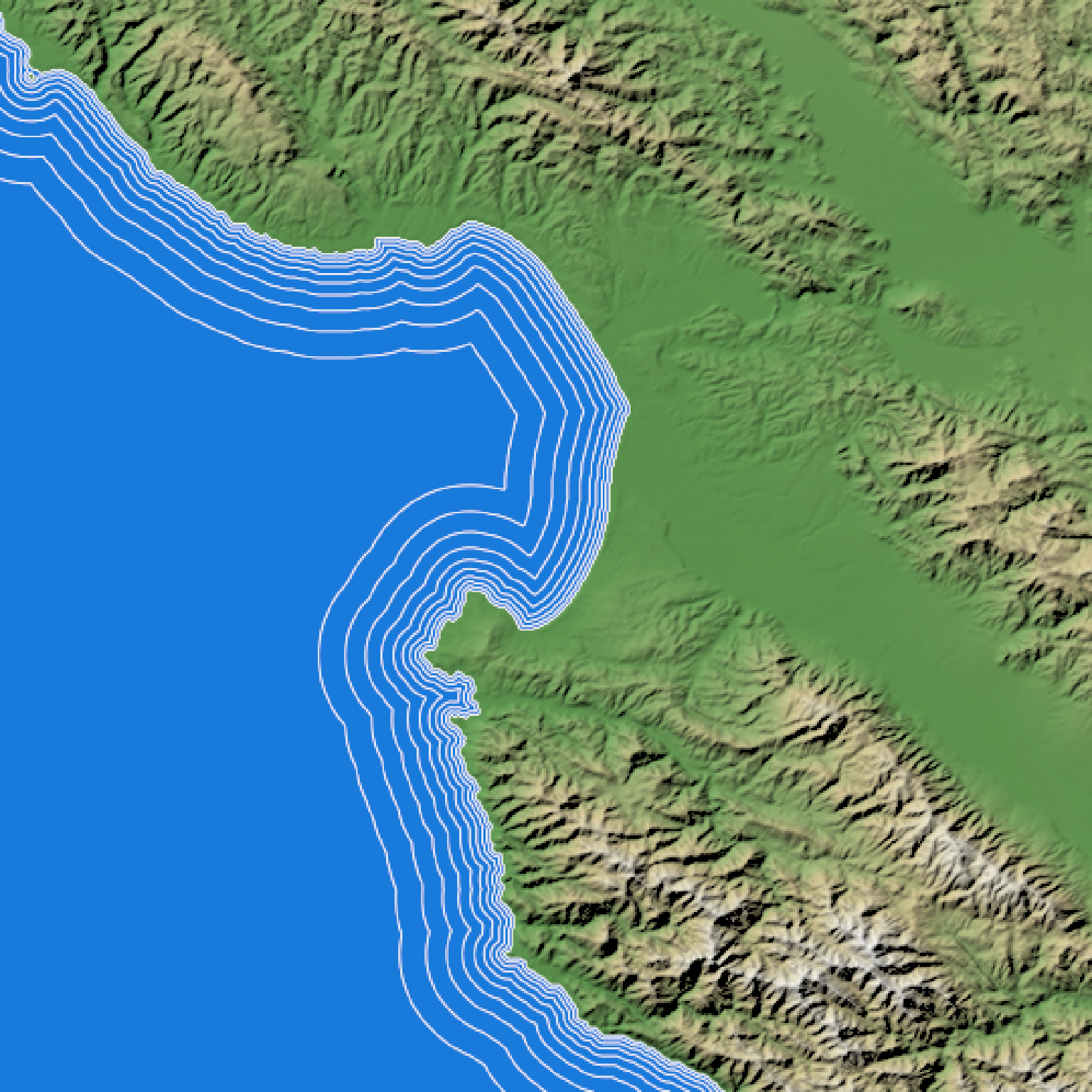if(run_documentation()) {
basemap %>%